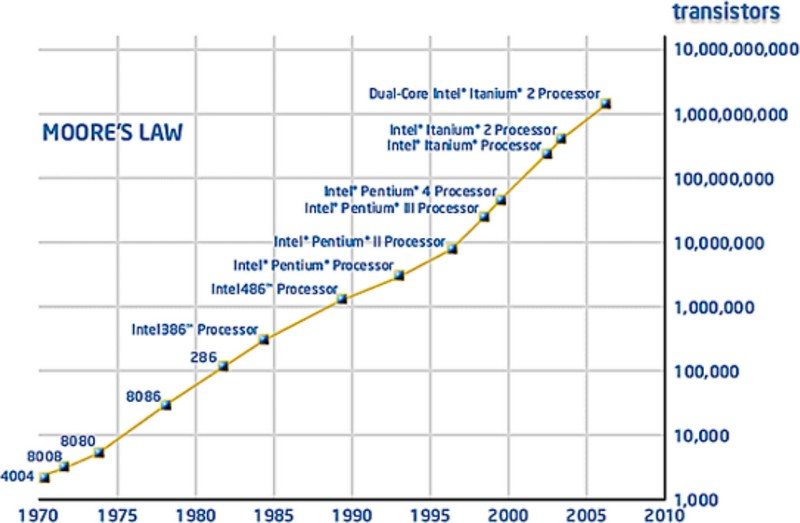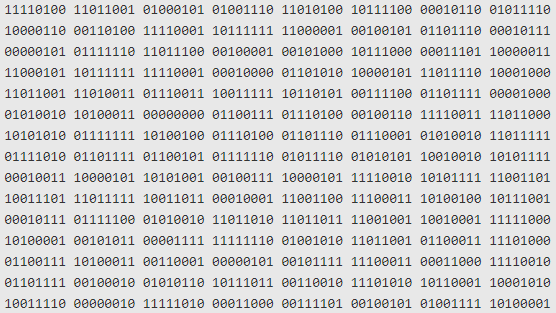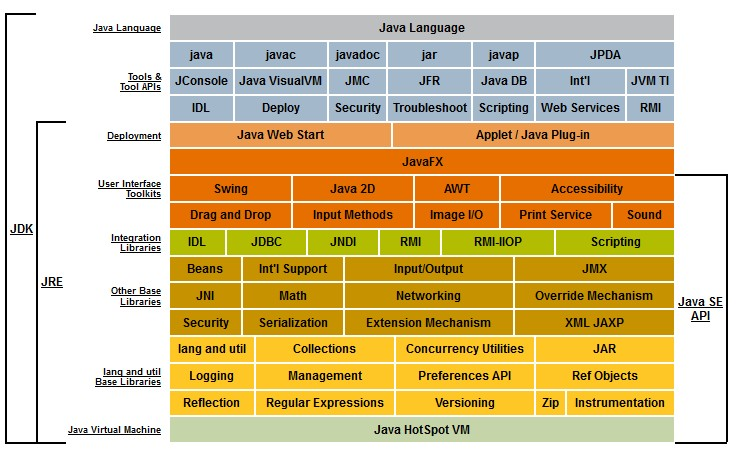# Computer Storage - Integer Data Type (Binary Data)

In computer, integer are stored in word from 8 to 64 bit.

Because CPU manipulates integer data type, they are also sometime called binary data type.

Bit Length Two's complement signed Unsigned Float / Double
8 Int8 Uint8
16 Int16 Uint16
32 Int32 Uint32 Float32
64 BigInt64 BigUint64 Float64

## Example

The integer number 42 in bit representation and the number of integer element that it can contains (min and max) 1)

representation bit
two’s complement 8-bit 0010 1010
two’s complement 32-bit 0000 0000 0000 0000 0000 0000 0010 1010
packed binary-coded decimal (BCD) 0100 0010
32-bit IEEE-754 floating-point 0100 0010 0010 1000 0000 0000 0000 0000
64-bit IEEE-754 floating-point 0100 0000 0100 0101 0000 0000 0000 0000 0000 0000 0000 0000 0000 0000 0000 0000

## Data Type

In a programming language, integers are made available as primitive type generally under the name:

• integer: for the short version (java stores them in 32bit)
• long: for the longer version (longer than integer) (java stores them in 64bit) - Long is a data type that allows higher range of number than integer at the cost of a higher storage.

but you may store integer in:

## Size

Integer size is defined in computer by the number of bit used to store them.

They are generally bound to the size of the cpu word (allowing quick bit operation) while the language was defined.

The integer range is:

• at least $-2^{n − 1}$
• to $2^{n − 1} − 1$

where:

• n is the storage size in bit
• -1 because you need a bit to store the byte order (?)
Size (Bit) Minimum (Bit) Maximum
8 $-2^{7} = 128$ $2^{7}−1 = 127$
16 $-2^{15} = 32.768$ $2^{15}−1 = 32.767$
32 $-2^{31} = -2,147,483,648$ $2^{31}−1 = -2,147,483,647$
64 $-2^{63} = 9.223.372.036.854.775.808$ $2^{63}−1 = 9.223.372.036.854.775.807$

## Overflow - The storage limitation may cause programs to crash

For example, if a programmer using the C language incorrectly declares as int a variable that will be used to store values greater than $2^{15}−1$ , the program will fail on computers with 16-bit integers creating a overflow failure. That variable should have been declared in C as long, which has at least 32 bits on any computer.

## Documentation / Reference

Discover MoreBit - Binary Data (Structure)

Binary Data is a computer file that contains binary data (0 or 1) Binary data may be described: at the bit level (base 2) at the byte level (base 2 - 8 bit) at the hexadecimal level (base 16)...Byte Array (multi-byte word)

A byte array is any array of byte. Library represents them generally as a multi-byte word with the possibility to change the endianess. See for instance, the Array Buffer in Javascript To a integer...CPU Data Type - Signed integer

A CPU signed integer is a CPU type that encodes a negative or positif integer. unsigned integerCPU Data Type - Unsigned integers

A CPU unsigned integer is a CPU type that encodes a non-negative integer integer. signed integerComputer - Floating-point (Representation and usage)

Computer representations of floating point numbers typically use a form of rounding to significant figures, but with binary numbers. The number of correct significant figures is closely related to the...Computer Number - Float64 (64-bit or double precision) floating-point number

Float64 is a floating point number with a 64bit precision. Float64 is also known as: 64-bit floating-point values, double precision floating-point 64-bit IEEE-754 floating-point or just double...Java - Integer

integer data type in Java. int (or Integer) is the 32 bit implementation of an integer. Java can also store an integer on 64 bit with a long. An integer in java is a sub-class of number They are...
Javascript - Byte Array (ArrayBuffer / DataView)

To manipulate a byte array, in javascript, you have: a ArrayBuffer that represents an array: of fixed amount of bytes (one element = 1 byte) of binary element known as typed object : Int8 / Uint8:...
Javascript - Integer

Integer aren't implemented in javascript, only double exists. See With integers, you have to take care that all calculations fit within the range between –2^53 and 2^53, but you don’t have to worry...Logical Data Modeling - Key

A key refers to a attribute, or a group of attributes, which assumes a unique value for: each entity in a entity set. or relationship in a relationship set. keyidentifier... database primary...